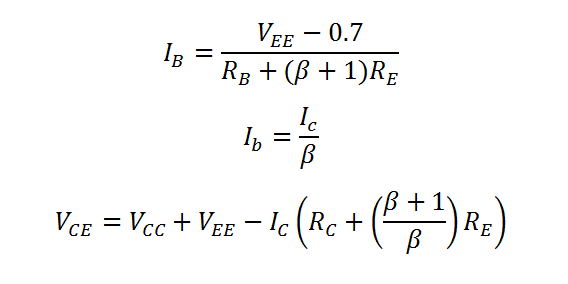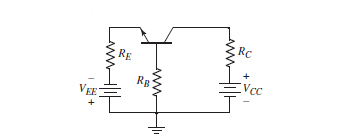# Common Base Amplifier Calculator (DC Analysis)

This calculator calculates the Base Current, Collector Current and Voltage between the collector and emitter, i.e. DC parameters for a BJT Common Base (CB) circuit. In this configuration the emitter of the BJT is the input, the collector is the output and the based is the common port, which is usually connected to ground. This configuration is equivalent to the common gate configuration in a FET transistor.

V
V
K-Ohm
K-Ohm
K-Ohm

#### Result

mA
mA
V